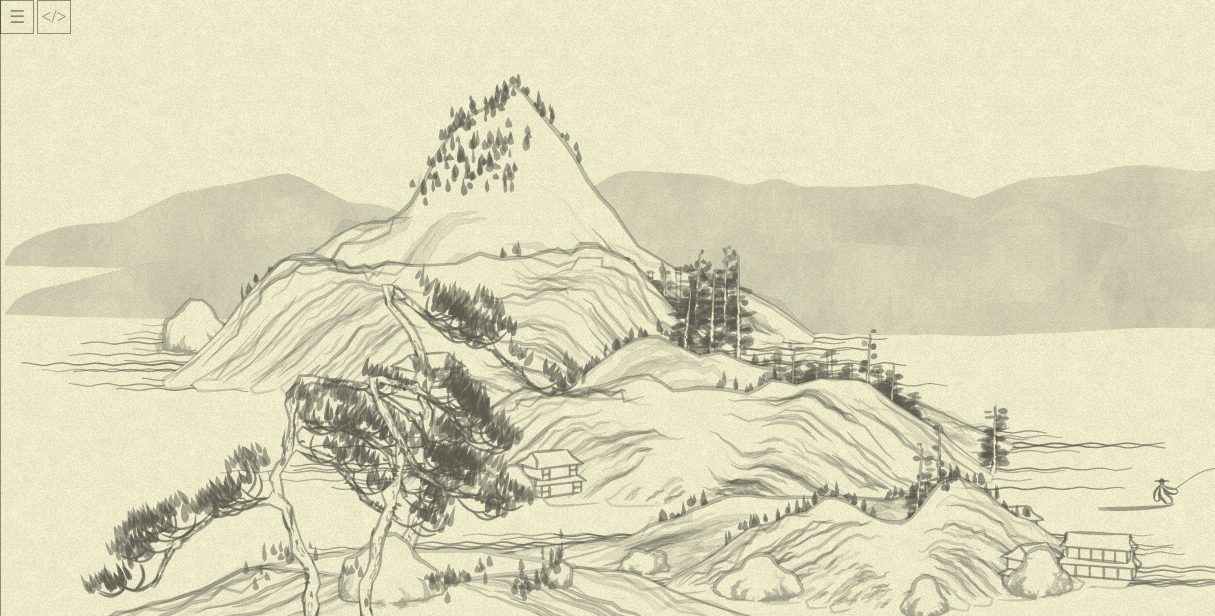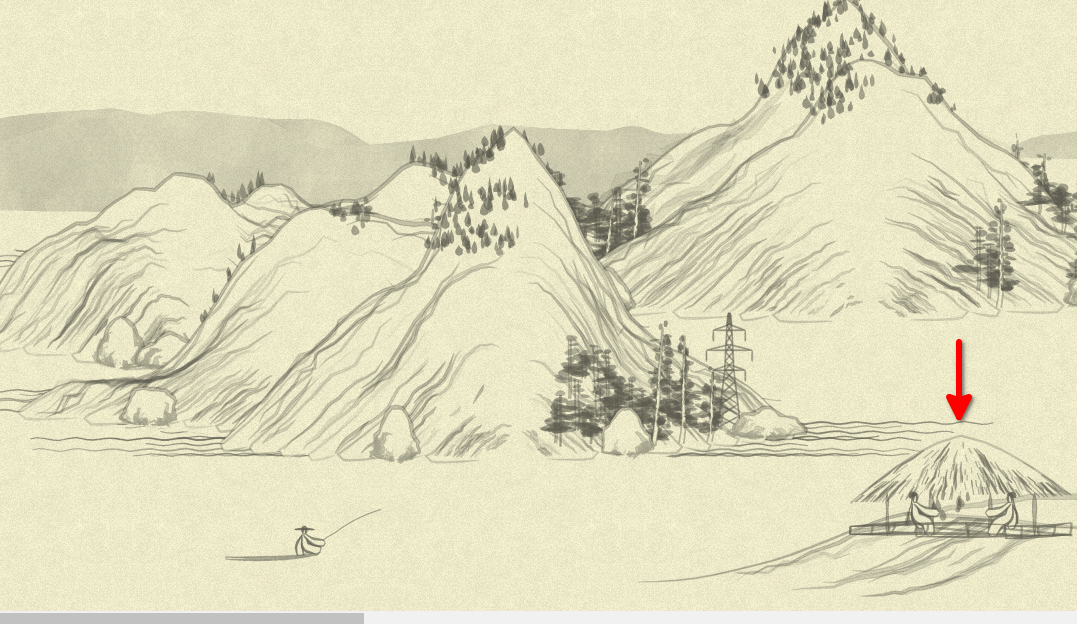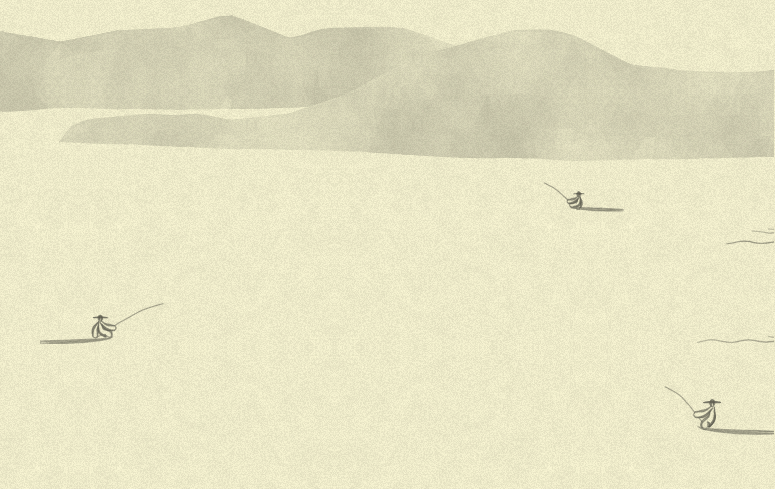Part of the Advent of Grok {Shan, Shui}*. See blog post for intro and table of contents.

« Day 13 | Day 15 »

• Commit: 6ede154
• Functions:
• Other days about:
• `mountplanner`: 1314

Yesterday we did quite a few bad things to `mountplanner`, can we go further, though?

First thing that bothers me is non-atomic operations with `MEM.planmtx`—which is, apparently, a global map of already occupied lines of the map. The repetitive operations I don’t like is regularly doing something like `MEM.planmtx[Math.floor(x / xstep)]`, so let’s OOP!

Analyzing the interface that is necessary to use `planmtx`, we can come with this:

``````/* Usage:
// Array of "is it occupied" data with step 5
occupied_points = new MountainMap(5)

// stores 40 zeroes corresponding to range 0-200
occupied_points.initRange(0, 200)

occupied_points.get(20)
occupied_points.isEmpty(20) // true if there is still 0

// increases by 1 all points corresponding to range 20-40
occupied_points.incRange(20, 40)
*/
class MountainMap {
constructor(step) {
this.data = []
this.step = step
}

get(at) { return this.data[Math.floor(at / this.step)] }
set(at, value) { this.data[Math.floor(at / this.step)] = value }
inc(at) { this.data[Math.floor(at / this.step)] += 1 }

isEmpty(at) { return this.get(at) == 0 }

initRange(from, to) {
range(Math.floor(from / this.step), Math.floor(to / this.step)).forEach( i => this.data[i] ||= 0 )
}
incRange(from, to) {
range(Math.floor(from / this.step), Math.floor(to / this.step)).forEach( i => this.data[i] += 1 )
}
}
``````

…and, replacing naked `planmtx` in code with this object, I am proudly presenting you with…Oh shoot.

It is just ranges definitions (+ 10 more mins of debugging, note `floor`/`ceil` change):

``````    initRange(from, to) {
range(Math.floor(from / this.step), Math.ceil(to / this.step)).forEach( i => this.data[i] ||= 0 )
}
incRange(from, to) {
range(Math.floor(from / this.step), Math.ceil(to / this.step)).forEach( i => this.data[i] += 1 )
}
``````

After that, the picture is back to the original (not repeating it, as usual! you just trust me).

Now, the `mountplanner` main body (with the `planmtx` renamed …and some other renames/cleanups) turns into this:

``````var xstep = 5;
var mountain_width = 200;

var x_range = range(xmin, xmax, xstep)

MEM.occupied_x ||= new MountainMap(xstep)
MEM.occupied_x.initRange(xmin, xmax)

x_range.forEach( x => {
var max_y = height_randomizer(x) * 480
range(0, max_y, 30).forEach( y => {
if (locmax(x, y, noise, 2)) {
var x_offset = x + rand(-500, 500);
var y_offset = y + 300;
if (chadd({ tag: "mount", x: x_offset, y: y_offset, h: noise(x, y) })) {
MEM.occupied_x.incRange(x_offset - mountain_width, x_offset + mountain_width)
}
}
})

if (Math.abs(x) % 1000 < Math.max(1, xstep - 1)) {
tag: "distmount",
x: x,
y: 280 - rand(50),
h: noise(x, max_y),
});
}
})

x_range.
filter( x => MEM.occupied_x.isEmpty(x) ).
forEach( x => chance(0.01, () => {
for (var y = 0; y < rand(4); y++) {
tag: "flatmount",
x: x + rand(-700, 700),
y: 700 - y * 50,
h: noise(x, y),
});
}
})
)

x_range.forEach( x =>
chance(0.2, () => chadd({ tag: "boat", x, y: 300 + rand(390) }, 400))
)

return reg;
``````

Now, I don’t like `chadd`. I like dynamic languages and prototype-based development (sometimes!) and this:

``````var registry = [];

registry.pushChunk = function(chunk, min_distance = 10) {
if(this.find( rchunk => Math.abs(rchunk.x - chunk.x) < min_distance )) {
return false
}
this.push(chunk);
return true;
}

// now, instead of chadd(...), we can `registry.pushChunk(...)`
``````

And, behold, the final `mountplanner`:

``````function mountplanner(xmin, xmax) {
function is_local_maximum(x, y, f, {vicinity}) {
var z0 = f(x, y);
if (z0 <= 0.3) {
return false;
}
for (var nx = x - vicinity; nx < x + vicinity; nx++) {
for (var ny = y - vicinity; ny < y + vicinity; ny++) {
if (f(nx, ny) > z0) {
return false;
}
}
}
return true;
}

var registry = [];

registry.pushChunk = function(chunk, min_distance = 10) {
if(this.find( rchunk => Math.abs(rchunk.x - chunk.x) < min_distance )) {
return false
}
this.push(chunk);
return true;
}

var samp = 0.03;
var noise = (x, y) => Math.max(Noise.noise(x * samp) - 0.55, 0) * 2;

var xstep = 5;
var mountain_width = 200;

var x_range = range(xmin, xmax, xstep)

MEM.occupied_x ||= new MountainMap(xstep)
MEM.occupied_x.initRange(xmin, xmax)

x_range.forEach( x => {
var max_y = Noise.noise(x * 0.01, Math.PI) * 480

range(0, max_y, 30).
filter( y => is_local_maximum(x, y, noise, {vicinity: 2}) ).
forEach( y => {
var x_offset = x + rand(-500, 500);
var y_offset = y + 300;
if (registry.pushChunk({ tag: "mount", x: x_offset, y: y_offset, h: noise(x, y) })) {
MEM.occupied_x.incRange(x_offset - mountain_width, x_offset + mountain_width)
}
})

if (Math.abs(x) % 1000 < xstep - 1) {
registry.pushChunk({
tag: "distmount",
x: x,
y: 280 - rand(50),
h: noise(x, max_y),
});
}
})

x_range.
filter( x => MEM.occupied_x.isEmpty(x) ).
forEach( x => chance(0.01, () => {
for (var y = 0; y < rand(4); y++) {
registry.pushChunk({
tag: "flatmount",
x: x + rand(-700, 700),
y: 700 - y * 50,
h: noise(x, y),
});
}
})
)

x_range.forEach( x =>
chance(0.2, () => registry.pushChunk({ tag: "boat", x, y: 300 + rand(390) }, 400))
)

return registry;
}
``````

Its retelling in human language would be:

• through all range of currently generated chunk
• …put a “mount(ain)” if it is a local maximum of the (smooth) Perlin noise
• …and mark the space around as occupied
• once in a thousand `x`s, put a “dist(ant )mount(ain)”
• after all mountains are generated, choose unoccupied points and (with 10% chance) place “flat( )mount(ains)” there
• ….and then, with 20% chance, put boats;
• also, there is a `registry` controlling nothing would be put at exactly same `x` line, this approach reduces possible “computerness” of the picture significantly.

BTW, while experimenting what would increasing boat chance lead to, I caught a nice picture: the arrow points to human figures I haven’t even knew this thing is able to generate! (Power tower is a nice touch too, but this one I seen already)…and in another side of the same picture, indeed, some boats (I increased chances to `1`):That’s … sweet little experiment. But back on track!

To close the gap between whole landscape planning and singular trees and houses, we need to cover all of those `"mount"/"flatmount"/...` tags processing—functions looking like `Mount.mountain(x, y, rand(i * 2))` which we’ve seen in day 12, and that’s what we’ll do tomorrow.

« Day 13 | Day 15 »